# 随笔

## 技术

2020-04-09   1.5k

# Machine Learning Week3

## 0x01 假设表示(Hypothesis Representation)

\begin{align*}& h_\theta (x) = g ( \theta^T x ) \newline \newline& z = \theta^T x \newline& g(z) = \dfrac{1}{1 + e^{-z}}\end{align*}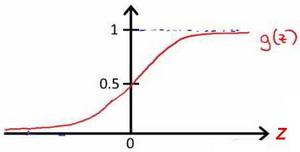hθ(x) 的作用是，对于给定的输入变量，根据选择的参数计算输出变量=1的可能性，即hθ(x) = P(y=0|x;θ) ，例如，如果对于给定的x，通过已经确定的参数计算得出hθ(x) = 0.7，则表示有70%的几率y为正向类，相应地为负向类的几率为1-0.7=0.3。

## 0x02 判定边界(Decision Boundary)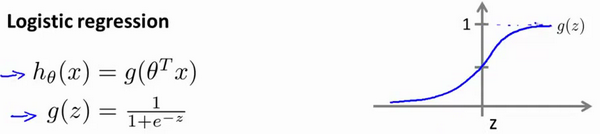z = 0时，g(z) = 0.5;

z > 0时，g(z) > 0.5;

z < 0时，g(z) < 0.5;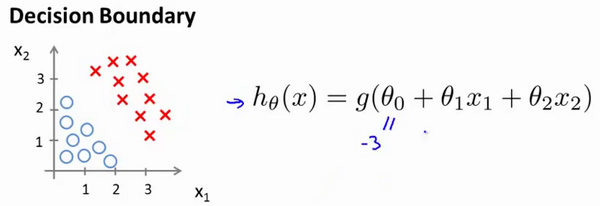## 0x03 代价函数(Cost Function)

\begin{align*}& J(\theta) = \dfrac{1}{m} \sum_{i=1}^m \mathrm{Cost}(h_\theta(x{(i)}),y{(i)}) \newline & \mathrm{Cost}(h_\theta(x),y) = -\log(h_\theta(x)) ; & \text{if y = 1} \newline & \mathrm{Cost}(h_\theta(x),y) = -\log(1-h_\theta(x)) ; & \text{if y = 0}\end{align*}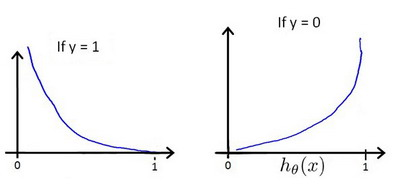$$\mathrm{Cost}(h_\theta(x),y) = - y ; \log(h_\theta(x)) - (1 - y) \log(1 - h_\theta(x))$$

$$J(\theta) = \dfrac{1}{m} \sum_{i=1}^m - y^{(i)} ; \log(h_\theta(x^{(i)})) - (1 - y^{(i)}) \log(1 - h_\theta(x^{(i)}))$$

\begin{align*} & h = g(X\theta)\newline & J(\theta) = \frac{1}{m} \cdot \left(-y{T}\log(h)-(1-y){T}\log(1-h)\right) \end{align*}

\begin{align*}& Repeat ; \lbrace \newline & ; \theta_j := \theta_j - \alpha \dfrac{\partial}{\partial \theta_j}J(\theta) \newline & \rbrace\end{align*}

\begin{align*} & Repeat ; \lbrace \newline & ; \theta_j := \theta_j - \frac{\alpha}{m} \sum_{i=1}^m (h_\theta(x^{(i)}) - y^{(i)}) x_j^{(i)} \newline & \rbrace \end{align*}

$$\theta := \theta - \frac{\alpha}{m}X^T(g(X\theta) - \vec y)$$

## 0x05 多分类(Muti-classification)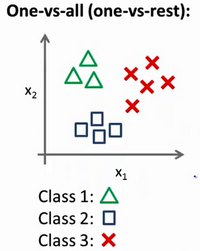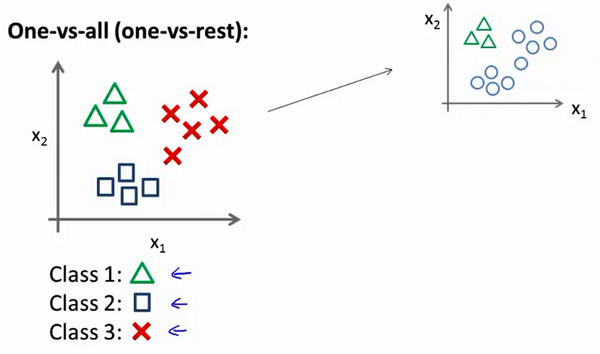$$\arg\max_\theta h_{\theta}^{(i)}$$

## 0x06 正则化(Regularization)

$$h_\theta (x) = \theta_0 + \theta_1 x_1 + \theta_2 x_2^2 + \theta_3 x_3^3 + \theta_4 x_4^4$$

$$min_\theta \frac{1}{2m}[\sum_{i=1}{m}(h_\theta(x{(i)})-y{(i)})2 + 1000\theta_3^2 + 1000\theta_4^2]$$

$$min_\theta \frac{1}{2m}[\sum_{i=1}{m}(h_\theta(x{(i)})-y{(i)})2 + \lambda\sum_{i=1}m\theta_j2]$$

### 正则化线性回归

\begin{align*} & \text{Repeat}\ \lbrace \newline & \ \ \ \ \theta_0 := \theta_0 - \alpha\ \frac{1}{m}\ \sum_{i=1}^m (h_\theta(x^{(i)}) - y{(i)})x_0{(i)} \newline & \ \ \ \ \theta_j := \theta_j - \alpha\ \left[ \left( \frac{1}{m}\ \sum_{i=1}^m (h_\theta(x^{(i)}) - y{(i)})x_j{(i)} \right) + \frac{\lambda}{m}\theta_j \right] &\ \ \ \ \ \ \ \ \ \ j \in \lbrace 1,2…n\rbrace\newline & \rbrace \end{align*}

\begin{align*}& \theta = \left( X^TX + \lambda \cdot L \right)^{-1} X^Ty \newline& \text{where}\ \ L = \begin{bmatrix} 0 & & & & \newline & 1 & & & \newline & & 1 & & \newline & & & \ddots & \newline & & & & 1 \newline\end{bmatrix}\end{align*}

### 正则化逻辑回归

$$J(\theta) = -\dfrac{1}{m} \sum_{i=1}^m [y^{(i)} ; \log(h_\theta(x^{(i)})) + (1 - y^{(i)}) \log(1 - h_\theta(x^{(i)}))] + \frac{\lambda}{2m}\sum_{j=1}^n \theta_j^2$$